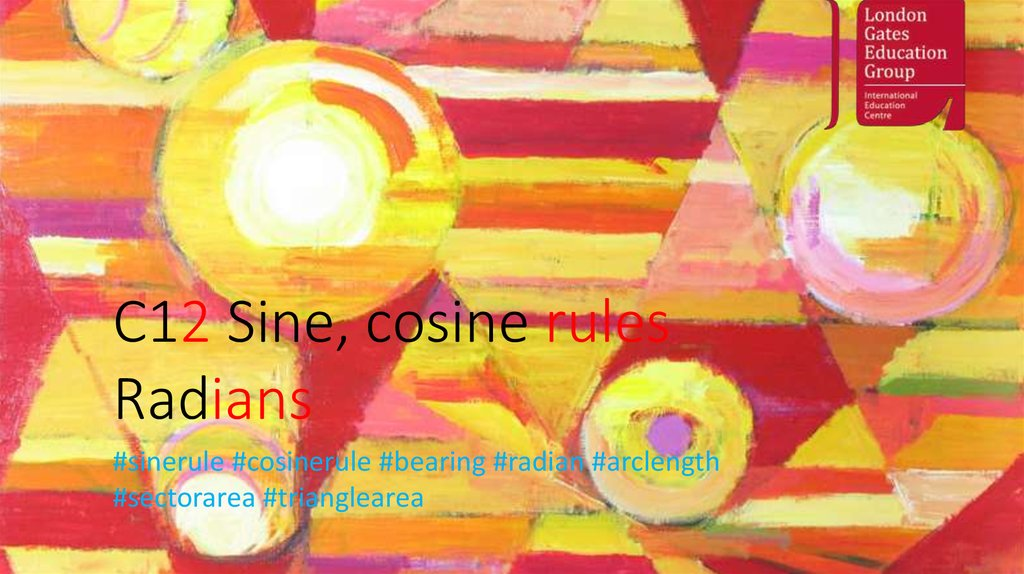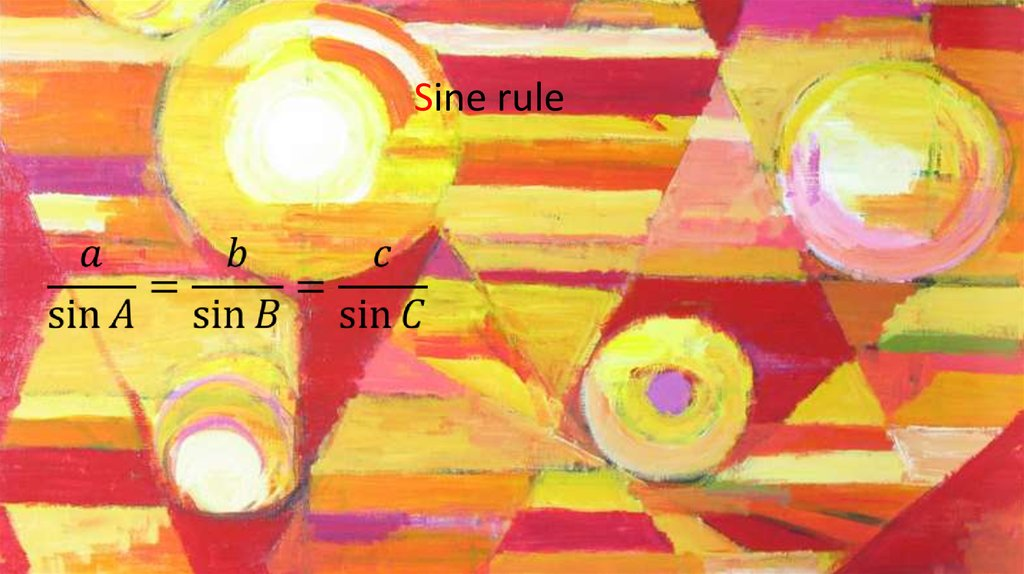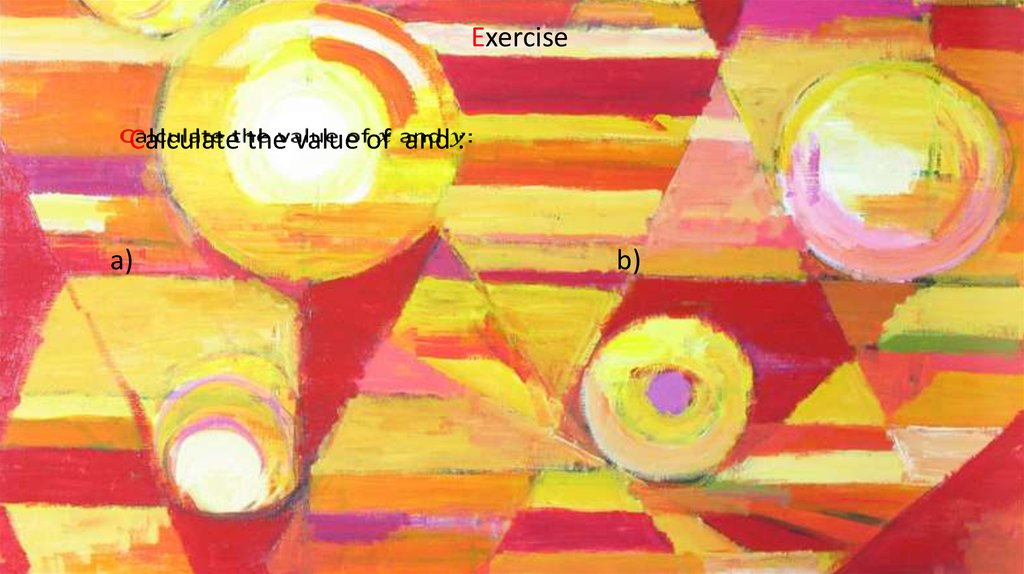# Sine, cosine, radians

## 1.

С12 Sine, cosine rules
Radians
#sinerule #cosinerule #bearing #radian #arclength
#sectorarea #trianglearea

Sine rule

## 3.

Exercise
Calculate the value of and :
a)
b)

## 4.

Exercise
Town is 6 km, on a bearing of 020, from town . Town is
located on a bearing of 055 from town and on a bearing of
120 from town .
Work out the distance of town from
a) town
b) town

Let us find :

## 6.

Exercise
Work out the value of
a)
b)

## 7.

Exercise
Work out the value of and
a)
b)

## 8.

• When the angle to be found is larger than the given angle
there are two possible results, because you can draw two
different triangles which satisfy the task
• These results and are connected:
In this example:
(to 3 s.f.)

## 9.

Exercise
Work out the possible values of and the corresponding
values of .

Cosine rule

## 11.

Exercise
Work out the value of the third side:

## 12.

Exercise
From a point a boat sails due north for 7 km to . The boat leaves and
moves on a bearing of 100 for 10 km until it reaches .
Calculate the distance of from .

## 13.

Exercise
Work out the size of the angle marked with * (to 3 s.f.):

## 14.

Exercise
A helicopter flies on a bearing of 080 from to , where km. It
then flies for 60 km to a point .
Given that is 80 km from calculate the bearing of from .

Data
1 side, 2 angles
2 sides, angle
2 sides, angle
3 sides
To find
side
angle
side
angle
Formula
Sine rule
Sine rule
Cosine rule
Cosine rule

Area

## 17.

Exercise
Work out the area of the given triangle:

## 18.

Exercise
Work out the value of :

## 19.

Angle is equal to 1 radian, when the length of arc is
equal to .

radians ,
so
radian

## 21.

The length of an arc
when is in radians.

## 22.

Exercise
The sector of the circle radius cm
contains an angle of radians. Find the
length of the arc, giving your answers in
the form , where and are integers.

## 23.

Exercise
Referring to the diagram, find:
a) The perimeter of the shaded region
when radians.
a) The value of when the perimeter of
the shaded region is 14 cm.

## 24.

Exercise
In the diagram is the diameter of a circle, centre and radius 2 cm. The
point lies on the circumference such that radians.
a) State the value, in radians, of .
The shaded region is a template for a
brooch.
(b) Find the exact value of the
perimeter of the brooch.

## 25.

The area of a sector
where is in radians

## 26.

Exercise
Work out the area of the shaded region:
(a)
(b)
(c)

## 27.

Exercise
The arc of a circle, centre and radius cm, is such that
radians.
Given that the perimeter of the minor sector is 30 cm:
(a) Calculate the value of .
(b) Show that the area of the minor sector is 36 .
(c) Calculate the area of the segment enclosed by the chord
and the minor arc .

## 28.

Exercise
The diagram shows a triangular plot of land. The sides , and have
lengths 12, 14 and 10 m respectively.
The lawn is a sector of a circle, centre
and radius 6 m.
(a) Show that to 3 s.f.
(b) Calculate the area of the flowerbed.

## 29.

Exercise
In the diagram, is the diameter of a circle, centre and
radius cm, and radians.
Given that the area of is equal to
that of the shaded segment,
show that

## 30.

Exercise (C2 Jan 2010)